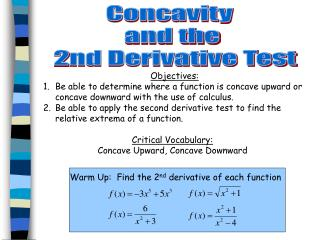Download PresentationObjectives: Be able to determine where a function is concave upward or concave downward with the use of calculus.

# Objectives: Be able to determine where a function is concave upward or concave downward with the use of calculus.

Download Presentation## Objectives: Be able to determine where a function is concave upward or concave downward with the use of calculus.

- - - - - - - - - - - - - - - - - - - - - - - - - - - E N D - - - - - - - - - - - - - - - - - - - - - - - - - - -
##### Presentation Transcript

1. Concavity and the 2nd Derivative Test • Objectives: • Be able to determine where a function is concave upward or concave downward with the use of calculus. • Be able to apply the second derivative test to find the relative extrema of a function. Critical Vocabulary: Concave Upward, Concave Downward Warm Up: Find the 2nd derivative of each function

2. Let f be differentiable on a open interval I. The graph of f is concave upward on I if f’(x) is increasing on the interval and concave downward on I if f’(x) is decreasing on the interval.

3. (0, ∞) (-∞,0) This is called a point of inflection. Concavity changes at these points. (-∞, 0) (2, ∞) (0, 2)

4. Let f be a function whose second derivative exists on an open interval I. 1. If f’’(x) > 0 for all x in I, then f is Concave Upward in I 2. If f’’(x) < 0 for all x in I, then f is Concave Downward in I

5. Example 1: Find the open intervals on which the following function is concave upward or concave downward. x = 1 and x = -1 These are called points of inflection. Concavity changes at these points. Concave Up: (-∞, -1) (1, ∞) Concave Down: (-1, 1) Interval (-∞, -1) (-1, 1) (1, ∞) Test Value x = -2 x = 0 x = 2 f’’(x) = (+) f’’(x) = (-) f’’(x) = (+) Sign of f’’(x) Conclusion Concave Up Concave Down Concave Up

6. Example 2: Find the open intervals on which the following function is concave upward or concave downward. Discontinuity: x = +/-2 Concave Up: (-∞, -2) (2, ∞) Concave Down: (-2, 2) Interval (-∞, -2) (-2, 2) (2, ∞) Test Value x = -3 x = 0 x = 3 f’’(x) = (+) f’’(x) = (-) f’’(x) = (+) Sign of f’’(x) Conclusion Concave Up Concave Down Concave Up

7. Page 342 #1-9 odds (MUST USE CALCULUS!!!!)

8. Let f be a function such that f’(c) = 0 and the second derivative of f exists on a open interval containing c. • If f’’(c) > 0, then f(c) is a relative minimum of f. 2. If f’’(c) < 0, then f(c) is a relative maximum of f. 3. If f’’(c) = 0, then the test fails. In such case, you use the First Derivative Test.

9. Example 3: Find the relative extrema for f(x) = -3x5 + 5x3 using the second derivative test. f’(x) = -15x4 + 15x2 Critical Numbers: x = 0, 1, -1 f(0) = 0: (0, 0) f(1) = 2: (1, 2) f(-1) = -2: (-1, -2) f’’(x) = -60x3 + 30x Inflection Points: x = 0 (-1, -2) (1, 2) (0, 0) Point Sign f’’(x) f’’(-1) > 0 f’’(0) = 0 f’’(1) < 0 Conclusion Relative Min Relative Max Test Fails Inflection

10. Example 4: Find the relative extrema for using the second derivative test. Critical Numbers: x = 0 f(0) = 1: (0, 1) Inflection Points: None (0, 1) Point Sign f’’(x) f’’(0) > 0 Conclusion Relative Min

11. Page 342 #11-33 odd, 45, 47 (MUST USE CALCULUS!!!!)